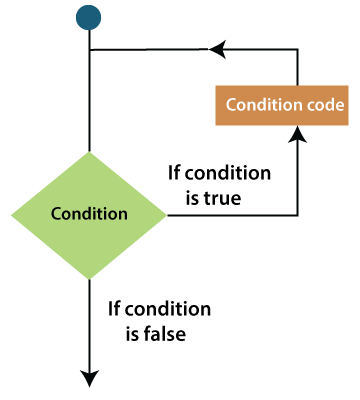# VBA Loops

There is a situation when you need to execute a block of code several numbers of times. In general, statements are performed in a predefined sequential.

The programming languages provide various control structures that allow for more complicated execution paths.

A loop statement allows you to execute a statement or group of statements multiple times. To get the most out of Excel and VBA, you know first how to use loops efficiently. The following image is the general form of a loop statement in VBA.For example: Consider a dataset and you want to highlight all the cells in even rows. You can use VBA loops to go through the range and analyze each cell row number. If it turns the even, then you give a color to it. Else you leave it as it is.

VBA provides the different types of loops to handle looping requirements. Below is the brief introduction of each loop, such as:

1. For loop: It executed a sequence of statements multiple times and compressed the code that manages the loop variable.

For loop uses a variable which cycle goes through a series of values within a specified range. The code inside the loop is then executed for each value in VBA.

The above For loop sets the variable i to have the values 1, 2, 3, …, 20, and for each of these values, run through the VBA code inside the loop. So, the loop adds each of the members of the array iArray to the variable.

2. For Each loop: It executes the block of code if there is at least one element that exists in the group and iterated for each element in a group.

For example: let’s listed every worksheet in the current excel workbook.

3. Do While loop: It executed as long as the condition is True or the loop should be repeated only when the situation is False.

4. Do Until loop: It will be executed as long as the condition is False or the loop should be repeated only when the situation is True.

### Loop control statements

Loop control statements can change execution from its normal sequence. When execution leaves a scope, all the remaining statements in the loop are NOT executed.

There are the following control statements supported by the VBA.

1. Exit for statement: It terminates the For loop statements and transfers the execution to the statement immaterially following the loop.
2. Exit do statement: It terminates the do-while statement and transfers the execution to the statement immediately following the loop.

Next TopicVBA For Loop

Previous articleVBA Data Types
Next articleCircular reference in Excel